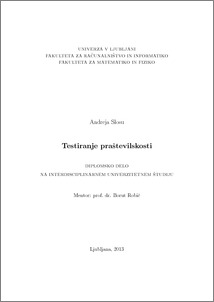# Primality testing

Andreja Slosu (2013) Primality testing. EngD thesis.Preview
PDF

## Abstract

With the boom in information technology and the penetration of these technologies in an increasing number of areas, such as electronic business, many questions about security arise. Data in electronic form bring many benefits, but they are vulnerable to various abuses. Therefore, data need to be adequately protected. For instance, when we perform a transaction over the Internet, our browser encrypts the credit card number. To do this, the browser usually uses encryption algorithms that are based on the theory of primes. For example: RSA asymmetric algorithm needs two large prime numbers p and q to calculate the module n. In the past, the primes were interesting because of their uniqueness. Euclid wrote the first definition of primes around 300 BC. The Sieve of Eratosthenes is the first known algorithm for deciding whether or not a number is a prime. In 1640, Fermat wrote his famous theorem to prove the primality of a number. The theorem is the basis of many subsequent algorithms. However, Fermat failed to prove the theorem, so that it was only later proved by Leibniz and Euler. My bachelor thesis gives an overview of algorithms for primality testing from antique times to the present. First of all, we give an overview of the naive algorithms that are simple but not suitable for large numbers. Then we describe probabilistic algorithms, i.e., algorithms that use random data. Next we describe the Solovay-Strassen and Miller-Rabin's algorithm and analyze their correctness and time complexity. The two algorithms are the most commonly used in practice. Even though these algorithms do not always return a correct answer, they are often used due to their speed. Next, we describe the deterministic algorithm AKS, discovered in 2002 by Agrawal, Kayal and Saxena. Although there have been several attempts, they are the first who succeeded to prove that the primality testing problem is in the class P of problems solvable in polynomial time. Their algorithm is a major achievement for theoretical computer science. Next, we describe the basic idea of the first version of the AKS algorithm and two improvements due to Lenstra-Pomerance and Bernstein. Finally, we describe in detail the sixth version of this algorithm from 2004.

Item Type: Thesis (EngD thesis)
Keywords: prime numbers, primality testing, AKS algorithm.
Number of Pages: 55
Language of Content: Slovenian
Mentor / Comentors:
Name and SurnameIDFunction
prof. dr. Borut Robič28Mentor